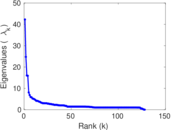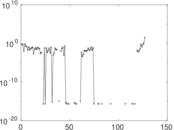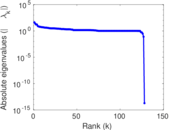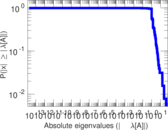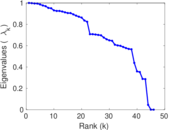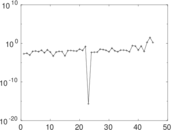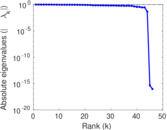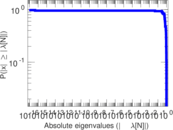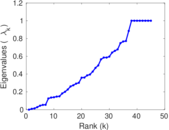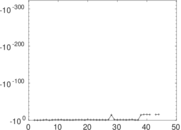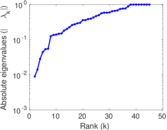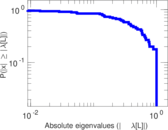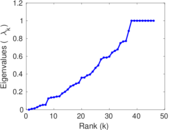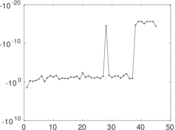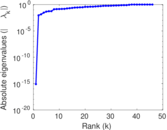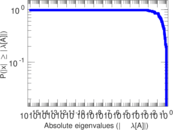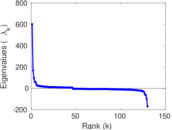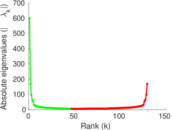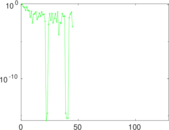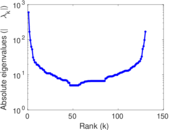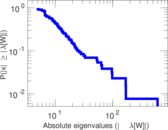# Wikibooks edits (mg)

This is the bipartite edit network of the Malagasy Wikibooks. It contains users and pages from the Malagasy Wikibooks, connected by edit events. Each edge represents an edit. The dataset includes the timestamp of each edit.

 Code `bmg` Internal name `edit-mgwikibooks` Name Wikibooks edits (mg) Data source http://dumps.wikimedia.org/ AvailabilityDataset is available for download Consistency checkDataset passed all tests Category Authorship network Dataset timestamp 2017-10-20 Node meaning User, article Edge meaning Edit Network formatBipartite, undirected Edge typeUnweighted, multiple edges Temporal dataEdges are annotated with timestamps

## Statistics

 Size n = 609 Left size n1 = 130 Right size n2 = 479 Volume m = 788 Unique edge count m̿ = 551 Wedge count s = 19,190 Claw count z = 1,075,709 Cross count x = 48,493,703 Square count q = 51 4-Tour count T4 = 78,342 Maximum degree dmax = 204 Maximum left degree d1max = 204 Maximum right degree d2max = 43 Average degree d = 2.587 85 Average left degree d1 = 6.061 54 Average right degree d2 = 1.645 09 Fill p = 0.008 848 56 Average edge multiplicity m̃ = 1.430 13 Size of LCC N = 362 Diameter δ = 18 50-Percentile effective diameter δ0.5 = 3.426 48 90-Percentile effective diameter δ0.9 = 7.558 28 Median distance δM = 4 Mean distance δm = 4.386 65 Gini coefficient G = 0.597 798 Balanced inequality ratio P = 0.271 574 Left balanced inequality ratio P1 = 0.211 929 Right balanced inequality ratio P2 = 0.371 827 Relative edge distribution entropy Her = 0.853 652 Power law exponent γ = 5.686 84 Tail power law exponent γt = 2.761 00 Tail power law exponent with p γ3 = 2.761 00 p-value p = 0.017 000 0 Left tail power law exponent with p γ3,1 = 1.941 00 Left p-value p1 = 0.000 00 Right tail power law exponent with p γ3,2 = 3.491 00 Right p-value p2 = 0.037 000 0 Degree assortativity ρ = −0.168 909 Degree assortativity p-value pρ = 6.761 65 × 10−5 Spectral norm α = 42.261 0 Algebraic connectivity a = 0.008 970 64 Spectral separation |λ1[A] / λ2[A]| = 1.708 80 Controllability C = 350 Relative controllability Cr = 0.579 470

## Plots

### Fruchterman–Reingold graph drawing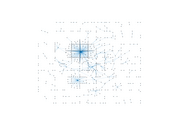### Degree distribution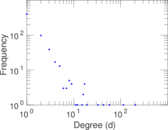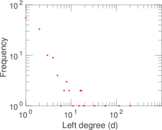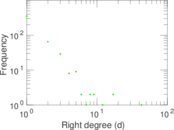### Cumulative degree distribution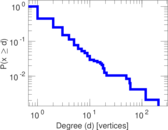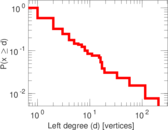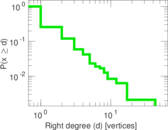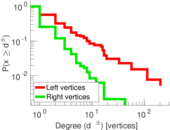### Lorenz curve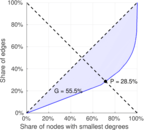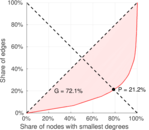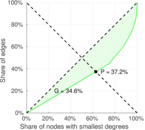### Spectral distribution of the adjacency matrix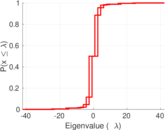### Spectral distribution of the normalized adjacency matrix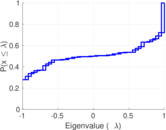### Spectral distribution of the Laplacian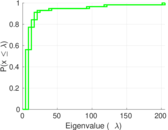### Spectral graph drawing based on the adjacency matrix### Spectral graph drawing based on the Laplacian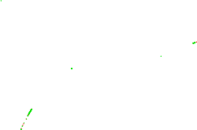### Spectral graph drawing based on the normalized adjacency matrix### Degree assortativity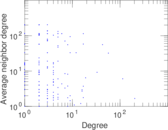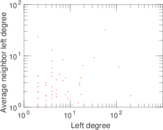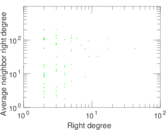### Zipf plot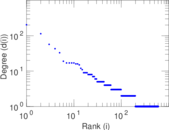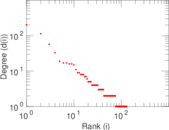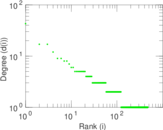### Hop distribution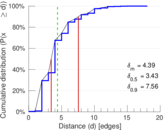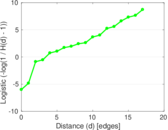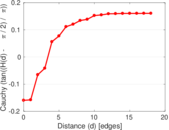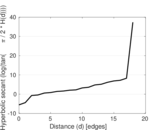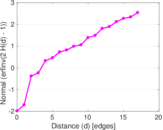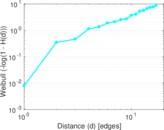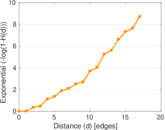### Double Laplacian graph drawing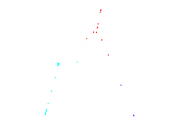### Delaunay graph drawing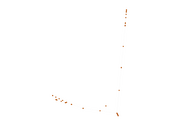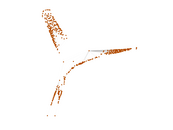### Edge weight/multiplicity distribution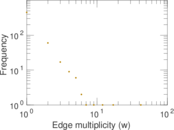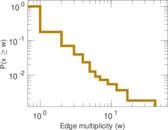### Temporal distribution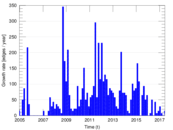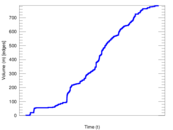### Temporal hop distribution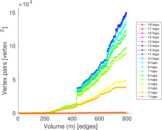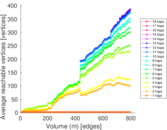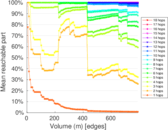### Diameter/density evolution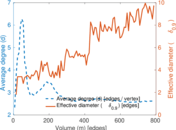### Matrix decompositions plots